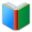# List 1 for 7th Grade MATH

Middle School Words: List 1 for 7th Grade MATH, all double sided cards of the list are in one page. You can download and print the worksheet from your browser directly.
 Free Online Vocabulary TestK12, SAT, GRE, IELTS, TOEFL
 Actions upon current list

 All lists of current grdae
Spelling Words:

Arts Words:

Social Words:

Math Words:

Science Words:

Literature Words:The Shoes of FortuneThe ShadowThe Adventures of Sherlock HolmesAdventures of Huckleberry Finn
 Worksheet - Middle School Words: List 1 for 7th Grade MATH

 Print: List 1 for 7th Grade MATH

Exam Word - https://www.examword.com/

Middle School Words: List 1 for 7th Grade MATH

 absolute value a real number regardless of its sign
 acute triangle a triangle whose interior angles are all acute
 bisector the line that divides something into two equal parts
 corresponding angles when two lines are crossed by another line, the angles in matching corners
 discount give reduction in price on; an amount or percentage deducted
 equilateral triangle a three-sided regular polygon
 experimental probability the ratio of the number of outcomes in which a specified event occurs to the total number of trials, not in a theoretical sample space but in an actual experiment.
 exponential notation scientific notation, a way of writing numbers that are too big or too small to be conveniently written in decimal form
 exterior angles the angle between a side of a rectilinear figure and an adjacent side extended outward
 interest the power of attracting or a fixed charge for borrowing money; usually a percentage of the amount borrowed
 interior angles the angle between adjacent sides of a rectilinear figure
 isosceles triangle a triangle with two equal sides
 negative less than zero; having a negative charge; reckoned in a direction opposite to that regarded as positive
 obtuse triangle a triangle that contains an obtuse interior angle
 outcome result; end or consequence; result of a random trial
 permutations rearrangement of the elements of a set
 positive involving advantage or good ; greater than zero; very sure; confident